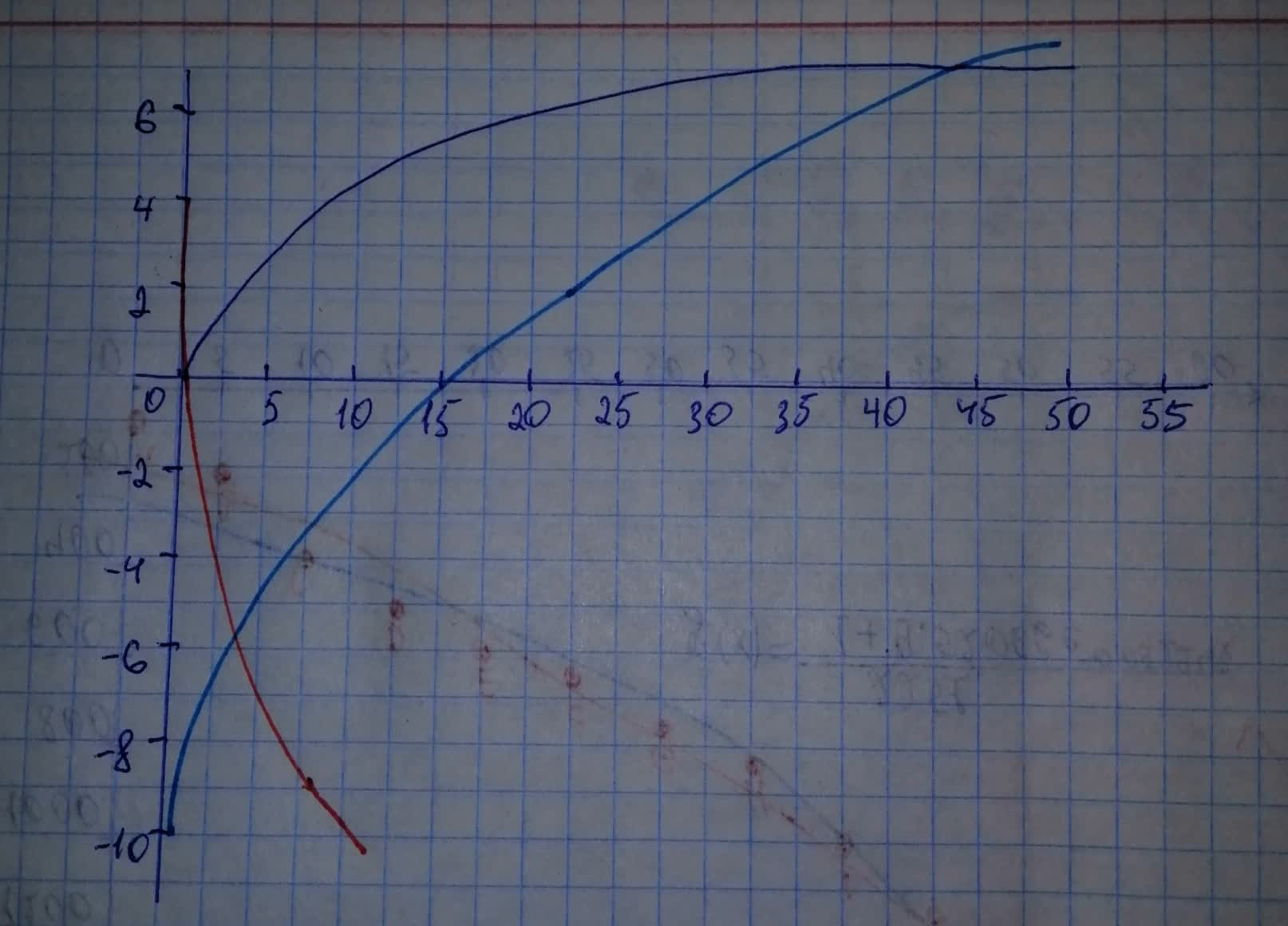# What transformations of the parent graph of f(x)=sqrt{x} produce the graphs of the following functions? a) m(x)=sqrt{7x - 3.5} - 10 b) j(x)=-2sqrt{12x} + 4hexacordoK 2021-01-06 Answered
What transformations of the parent graph of
$f\left(x\right)=\sqrt{x}$
produce the graphs of the following functions?
a)
b)
You can still ask an expert for help

• Questions are typically answered in as fast as 30 minutes

Solve your problem for the price of one coffee

• Math expert for every subject
• Pay only if we can solve itirwchh
Step 1
First we are going to graph the prent function and the first transformation and second transformation of parent function.Step 2
As we can see from the graph the first transformation of parent function has horizontal shift of 0.5 units to the right, and vertical shift of 10 units down. Vertical stretch was also preformed on the first function.
The second transformation of parent function has been reflected across x-axis and vertically shifted up 4 units. Horizontal compression was also preformed on the for the second function.